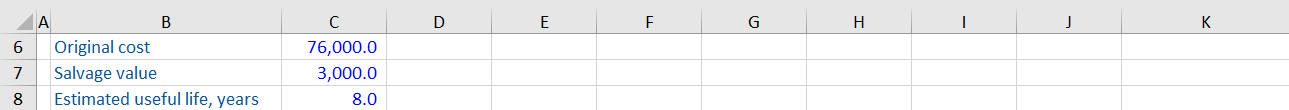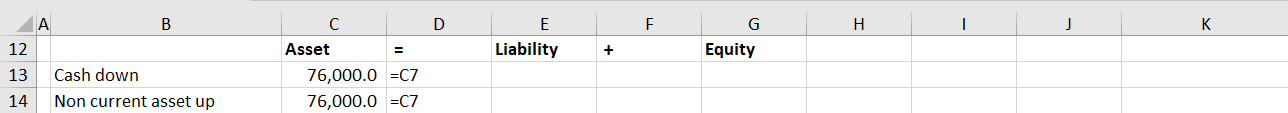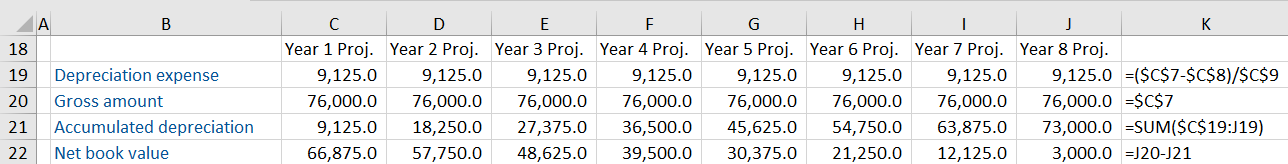## What is Accumulated Depreciation?

Accumulated depreciation is the total depreciation expense allocated to a long-term tangible asset since it was put into use and represents the total value by which a company’s assets have been depreciated. In other words, it is the amount of cost allocated to depreciation expenditure so far in the useful life of an asset.

Depreciation is the process of reducing the value of assets in the balance sheet by transferring part of it to the profit and loss account for each period.

## Key Learning Points

• Depreciation is a non-cash expense and represents the consumption of benefits of a tangible asset over time
• The value of an asset on the balance sheet is reported at net book value (NBV) which is calculated as cost of asset minus accumulated depreciation
• There are many depreciation methods used which each have different impacts on the income statement but the same overall economic cost to the company
• Further details on the depreciation policy used can be found in the financial footnotes, which also provides a breakdown of property, plant and equipment (PP&E) net

## Accounting for Accumulated Depreciation

The accumulated depreciation account is a contra asset account that reduces the book value of the assets reported on the balance sheet. As the depreciation expense is recognized in profits, the accumulated depreciation balance increases.

Accumulated depreciation subtracted from the cost of an asset is equal to the asset’s net book value.

The depreciation expense for the year is the portion of the initial cost of a company’s fixed asset allocated to a single period. Depreciation expenses are recognized on the income statement as non-cash expenses which decreases the company’s net income.

There are two main methods used to calculate depreciation: the straight-line method and reducing balance method.

The straight-line method expenses the same depreciation charge for each year of a tangible asset’s life. The future benefits of the asset are expensed at the same rate each year.

For the reducing balance method, the amount of depreciation charged to an asset declines each year because the same fixed percentage is applied to the falling value yearly. As a result, we never get an asset value down to zero until it is scrapped.

## Depreciation Calculation

A business purchases a machine for cash. How will the purchase be reflected in the balance sheet?The machine is purchased using cash so the cash balance is reduced. The transaction results in a corresponding increase the non-current asset account.It is now the start of Year 1. Show the forecast schedule below:The first step is to calculate the depreciation expense. In this example, the company is using a straight line depreciation method. This is calculated as the cost of the asset less its salvage value, divided by the number of years the asset is in service. The straight line depreciation method charges the same expense for each year, so this calculation can be copied across.

Tip: Type F4 on your keyboard when editing a formula to lock cells. This applies the dollar \$ signs to the cell.

The gross amount is equal to the cost of the asset and remains unchanged.

Accumulated depreciation is calculated as the sum of the current and prior year’s depreciation expense. Locking to cell C19 adds the depreciation expense incurred in year 1 to the depreciation incurred up to the projected year in question.

Finally, the net book value can be calculated. The net book value is the value the asset is reported at in the balance sheet and is calculated as the cost less accumulated depreciation.

We only schedule up to year 8 as this is the number of years the asset is in service i.e. its useful life. You will notice that the net book value reported in the final year (year 8) is equal to the salvage value of the asset.

## Accumulated Depreciation Calculation

Accumulated depreciation is calculated as follows:

(Gross cost of Asset – Salvage value)  / Useful life) x Number of years in use

A company purchased mining equipment at the beginning of year 0 for \$500,000. The equipment has a residual value of \$100,000 and an expected useful life of 5 years. Calculate the accumulated depreciation account balance (straight line) as on year 3.

The accumulated depreciation calculation is:All figures are in (\$) thousands